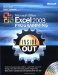# Chapter 1: What s New in Excel 2003

 < Day Day Up >

Microsoft Excel has been around since 1985, so it's no surprise that the basic spreadsheet elements of the program have remained fairly constant for quite some time. That said, there is always room for improvement, and Microsoft Office Excel 2003 has a bunch of new features that make data gathering, collaboration, and data transfer much more effective than in previous versions. The bulk of the improvements in Excel 2003 revolve around the use of Extensible Markup Language, or XML, which is a flexible, text-based markup system that lets you describe the contents of a spreadsheet so that the data it contains can be handled automatically instead of manually, saving time and reducing the possibility of errors introduced from re-keying or copying the data. Excel 2003 also comes with a suite of improved statistical functions, which are far more precise than in previous versions of the program.

## Using Improved Statistical Functions

Most Excel users will never go beyond the relatively simple formulas and formatting tasks used to track business and financial data, but scientific and advanced business or academic users who take advantage of the advanced statistical functions available in Excel need great precision from those functions. The Excel programming team changed how the program calculates the results of quite a few statistical functions, improving the accuracy of those results and making them much more useful to advanced users. Table 1-1 lists the enhanced functions and describes each one.

 BINOMDIST Determines the probability that a set number of true/false trials, where each trial has a consistent chance of generating a true or false result, will result in exactly a specified number of successes (for example, exactly five out of ten coin flips will end up heads). CHIINV Finds a value that best fits a result in a chi-squared distribution. CONFIDENCE Returns a value you can use to construct a confidence interval for a population mean. CRITBINOM Determines when the number of failures in a series of true/false trials exceeds a criterion (for example, more than 5 percent of light bulbs in a production run fail to light). DSTDEV Estimates the standard deviation of values in a column by considering only those values that meet a criterion. DSTDEVP Calculates the standard deviation of values in a column based on every value in the column. DVAR Estimates the variance of values in a column or list by considering only those values that meet a criterion. DVARP Calculates the variance of values in a column or list based on every value in the column. FINV Returns the value that would generate a target result from an F-test (a test of variability between two data sets). FORECAST Calculates future values based on an existing time series of values. GAMMAINV Returns the value that would generate a given result from a gamma- distributed (that is, skewed) data set. GROWTH Predicts the exponential growth of a data series. HYPGEOMDIST Returns the probability of selecting an exact number of a single type of item from a mixed set of objects. For example, a jar holds 20 marbles, 6 of which are red. If you choose three marbles, what is the probability you will pick exactly one red marble? INTERCEPT Calculates the point at which a line will intersect the y-axis. LINEST Generates a line that best fits a data set by generating a two- dimensional array of values to describe the line. LOGEST Generates a curve that best fits a data set by generating a two- dimensional array of values to describe the curve. LOGINV Returns the inverse logarithm of a value in a distribution. LOGNORMDIST Returns the number of standard deviations a value is away from the mean in a lognormal distribution. NEGBINOMDIST Returns the probability that there will be a given number of failures before a given number of successes in a binomial distribution. NORMDIST Returns the number of standard deviations a value is away from the mean in a normal distribution. NORMINV Returns a value that reflects the probability a random value selected from a distribution will be above it in the distribution. NORMSDIST Returns a standard normal distribution, with a mean of 0 and a standard deviation of 1. NORMSINV Returns a value that reflects the probability a random value selected from the standard normal distribution will be above it in the distribution. PEARSON Returns a value that reflects the strength of the linear relationship between two data sets. POISSON Returns the probability of a number of events happening, given the Poisson distribution of events. RAND Generates a random value. RSQ Returns the square of the Pearson coefficient of two sets of values. SLOPE Returns the slope of a line. STDEV Estimates the standard deviation of a numerical data set based on a sample of the data. STDEVA Estimates the standard deviation of a data set (which can include text and true/false values) based on a sample of the data. STDEVP Calculates the standard deviation of a numerical data set. STDEVPA Calculates the standard deviation of a data set (which can include text and true/false values). STEYX Returns the predicted standard error for the y value for each x value in a regression. TINV Returns a t value based on a stated probability and degrees of freedom. TREND Returns values along a trend line. VAR Estimates the variance of a data sample. VARA Estimates the variance of a data set (which can include text and true/ false values) based on a sample of the data. VARP Calculates the variance of a data population. VARPA Calculates the variance of a data population, which can include text and true/false values. ZTEST Returns the probability that the mean of a data sample would be greater than the observed mean of data in the set.

 < Day Day Up >Microsoft Office Excel 2003 Programming Inside Out (Inside Out (Microsoft))
ISBN: 0735619859
EAN: 2147483647
Year: 2006
Pages: 161

Similar book on Amazon